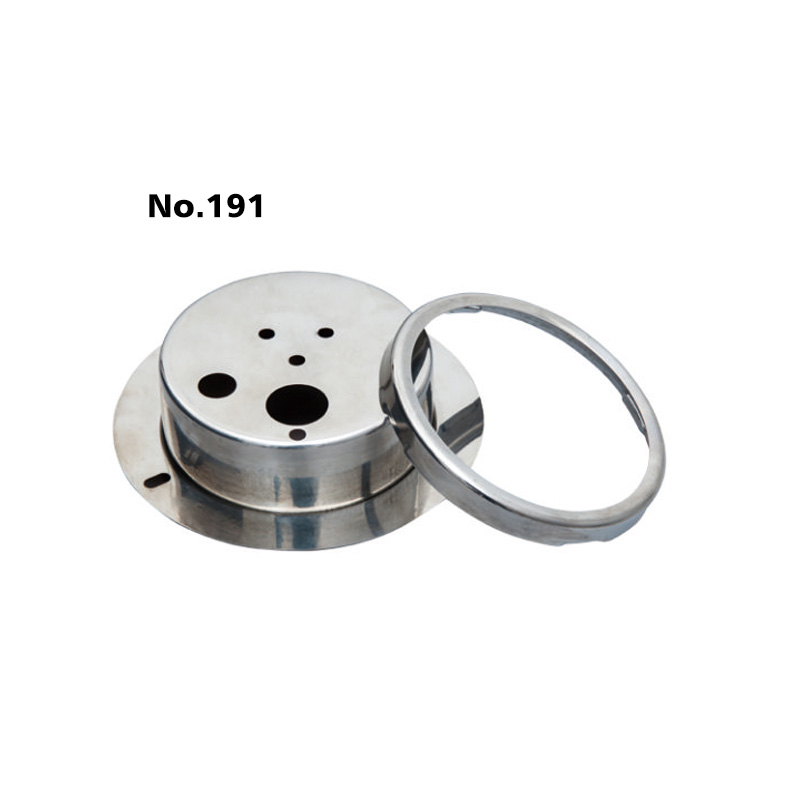# The Differential Pressure Of Pressure Gauge

Update:24 Jul 2020
Summary:

Pressure gauge, instrument for measuring the condition […]

Pressure gauge, instrument for measuring the condition of a fluid (liquid or gas) that is specified by the force that the fluid would exert, when at rest, on a unit area, such as pounds per square inch or newtons per square centimetre.The reading on a gauge, which is the difference between two pressures, is known as the gauge pressure. If the lower of the pressures is the pressure of the atmosphere, the total, or absolute, pressure is the sum of the gauge and atmospheric pressures.

The simplest device for measuring static pressures up to about 90 pounds per square inch (62 newtons per square cm) is a U-tube manometer (shown in the figure), in which one column of a liquid in the tube is open to a region of high pressure and the other column to a region of low pressure.

The differential pressure is indicated by the difference in level between the two columns of liquid, and it is calculated as the difference in level multiplied by the density of the liquid. The manometer liquids most commonly used are mercury, oil, alcohol, and water.